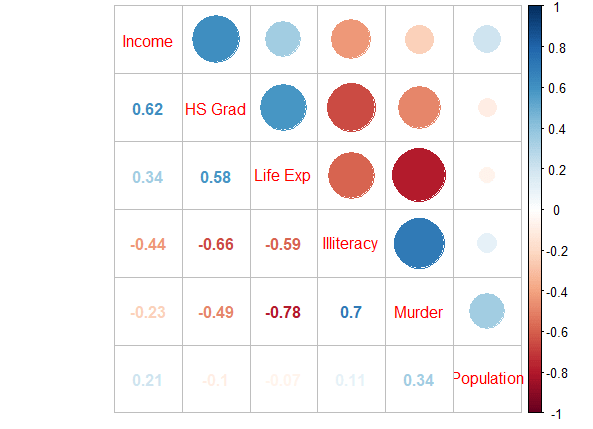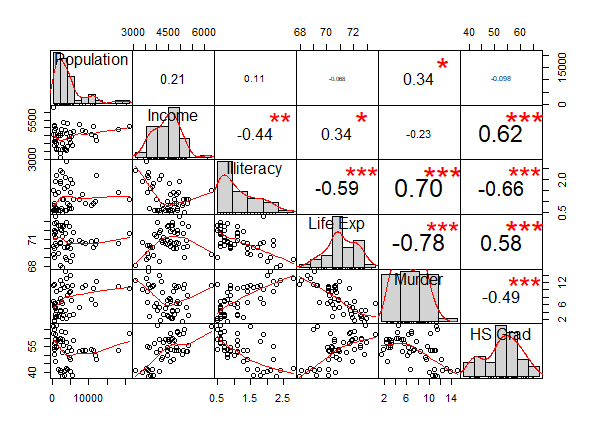## R语言 相关性分析

#### 协方差和相关系数

X, Y是两个随机变量
μx, μy是两个随机变量的均值

• Spearman相关系数
• Kendall的τ系数
• γ系数

Spearman相关系数平时可能用的比较多，其思路是分别求出每个变量各自排序后的秩次，然后将秩次作为变量，从而计算这个秩次变量的Pearson相关系数（用的还是Pearson的计算公式）

#### 相关系数检验

• 两个独立样本之间的比较：如男性中体重与血压的相关系数r1和女性中体重与血压的相关系数r2的比较
• 同一样本中的两个相关系数的比较：如体重与血压的相关系数r1和体重与血糖的相关系数r2的比较

#### R语言计算相关性以及显著性检验

states <- state.x77[,1:6]


> cor(x = states[,"Population"], y = states[,"Income"], use = "everything", method = "pearson")
 0.2082276


> cor(states)
Population     Income Illiteracy    Life Exp     Murder     HS Grad
Population  1.00000000  0.2082276  0.1076224 -0.06805195  0.3436428 -0.09848975
Income      0.20822756  1.0000000 -0.4370752  0.34025534 -0.2300776  0.61993232
Illiteracy  0.10762237 -0.4370752  1.0000000 -0.58847793  0.7029752 -0.65718861
Life Exp   -0.06805195  0.3402553 -0.5884779  1.00000000 -0.7808458  0.58221620
Murder      0.34364275 -0.2300776  0.7029752 -0.78084575  1.0000000 -0.48797102
HS Grad    -0.09848975  0.6199323 -0.6571886  0.58221620 -0.4879710  1.00000000


cor函数的method参数支持的相关性可选类型除了pearson外，还有spearman和kendall，如：

> cor(x = states[,"Population"], y = states[,"Illiteracy"], method = "spearman")
 0.3130496


> cor.test(x = states[,"Population"], y = states[,"Income"])

Pearson's product-moment correlation

data:  states[, "Population"] and states[, "Income"]
t = 1.475, df = 48, p-value = 0.1467
alternative hypothesis: true correlation is not equal to 0
95 percent confidence interval:
-0.07443435  0.45991855
sample estimates:
cor
0.2082276


res <- cor.test(x = states[,"Population"], y = states[,"Income"])
res$p.value res$conf.int


> corr.test(states, use = "complete", method = "pearson", adjust = "none")
Call:corr.test(x = states, use = "complete", method = "pearson",
Correlation matrix
Population Income Illiteracy Life Exp Murder HS Grad
Population       1.00   0.21       0.11    -0.07   0.34   -0.10
Income           0.21   1.00      -0.44     0.34  -0.23    0.62
Illiteracy       0.11  -0.44       1.00    -0.59   0.70   -0.66
Life Exp        -0.07   0.34      -0.59     1.00  -0.78    0.58
Murder           0.34  -0.23       0.70    -0.78   1.00   -0.49
HS Grad         -0.10   0.62      -0.66     0.58  -0.49    1.00
Sample Size
 50
Probability values (Entries above the diagonal are adjusted for multiple tests.)
Population Income Illiteracy Life Exp Murder HS Grad
Population       0.00   0.15       0.46     0.64   0.01     0.5
Income           0.15   0.00       0.00     0.02   0.11     0.0
Illiteracy       0.46   0.00       0.00     0.00   0.00     0.0
Life Exp         0.64   0.02       0.00     0.00   0.00     0.0
Murder           0.01   0.11       0.00     0.00   0.00     0.0
HS Grad          0.50   0.00       0.00     0.00   0.00     0.0


res <- corr.test(states, use = "complete", method = "pearson", adjust = "none")
res$r res$ci


#### 可视化

library(corrplot)
res_cor <- cor(states)
corrplot(corr=res_cor)corrplot(corr = res_cor,order = "AOE",type="upper",tl.pos = "d")
corrplot(corr = res_cor,add=TRUE, type="lower", method="number",order="AOE",diag=FALSE,tl.pos="n", cl.pos="n")chart.Correlation(states, method = "pearson")